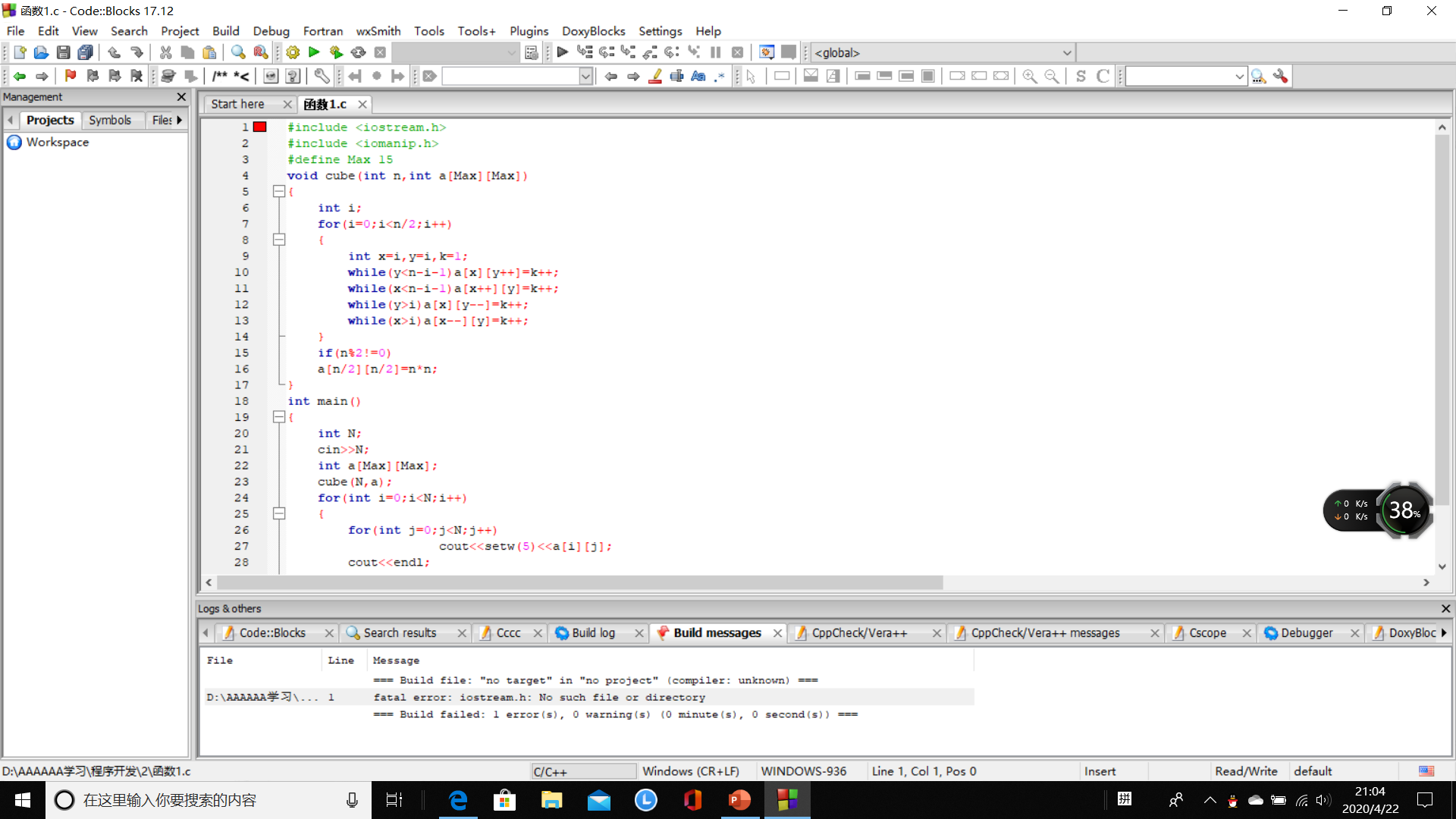2020-04-22 21:08

# 输入一个自然数Ｎ（N属于2到15），要求输出如下的魔方阵，即边长为N*N，元素取值为1至N*N，1在左上角，呈顺时针方向依次放置各元素。

【问题描述】

N=3时：
1 2 3
8 9 4
7 6 5
【输入形式】

【输出形式】

【输入样例】
4
【输出样例】
1 2 3 4
12 13 14 5
11 16 15 6
10 9 8 7``````#include <iostream.h>
#include <iomanip.h>
#define Max 15
void cube(int n,int a[Max][Max])
{
int i;
for(i=0;i<n/2;i++)
{
int x=i,y=i,k=1;
while(y<n-i-1)a[x][y++]=k++;
while(x<n-i-1)a[x++][y]=k++;
while(y>i)a[x][y--]=k++;
while(x>i)a[x--][y]=k++;
}
if(n%2!=0)
a[n/2][n/2]=n*n;
}
int main()
{
int N;
cin>>N;
int a[Max][Max];
cube(N,a);
for(int i=0;i<N;i++)
{
for(int j=0;j<N;j++)
cout<<setw(5)<<a[i][j];
cout<<endl;
}
return 0;
}

```求大佬帮忙找出哪错了？
``````
• 写回答
• 关注问题
• 收藏
• 邀请回答